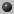Some useful links that I use everyday or every time I observe....

# Astrophysical Constantslook also in:Physical constants from NIST

Exponent (base 10) of decimal numbers: e n = 10^n . Units are separated by spaces, positive numbers denote powers, all units to the right of the slash are in the denominator (negative powers).

## AstronomyDistances

``` 1 AU (Astronomical Unit) = 1.496e11 m = 1.496e13 cm
1 pc (parsec)            = 3.086e16 m = 3.086e18 cm
= 206265 AU
= 3.26 Light years
```Sun

``` Radius               6.960e8 m     = 6.960e10 cm
Mass                 1.989e30 kg   = 1.989e33 g
Luminosity           3.85e26 W     = 3.85e33 erg
Surface gravity      2.736e2 m/s2  = 2.736e4 cm/s2
Temperature          5780 K

Absolute magnitude   5.72 mag (U)
5.54 mag (B)
4.87 mag (V)
4.74 mag (bolometric)
```Magnitudes

```   mag1-mag2 = -2.5 log(flux_1/flux_2)

-(mag1-mag2)    1     2.5   5   10   15   20
flux_1/flux_2    2.51  10    1e2 1e4  1e6  1e8

-(mag1-mag2) << 1 :  -(mag1-mag2)+1 = flux_1/flux_2

```Absolute calibration of photometry

```  Filter lambda   flux density for 0 mag    A/A_v
band    nm      W/(m^2 hz^1)   W/(nm m^2)

U       365      1.81e-23      4.22e-11   1.531
B       440      4.26e-23      6.40e-11   1.324
V       550      3.63e-23      3.75e-11   1.000
R       720      2.94e-23*     1.70e-11   0.748
I       900      2.24e-23*     0.83e-11   0.482
Rc      640      3.08e-23      2.25e-11*  0.830
Ic      790      2.55e-23      1.22e-11*  0.628
J      1250      1.60e-23*     3.07e-12   0.282
H      1650      1.09e-23*     1.20e-12   0.175
K      2200      6.49e-24      4.10e-13   0.112
L      3500      2.61e-24*     6.40e-14   0.058
M      4800      1.46e-24*     1.90e-14   0.023
u       350      1.33e-23*     3.25e-11
v       410      4.03e-23*     7.18e-11
b       470      4.28e-23*     5.81e-11
y       550      3.73e-23*     3.70e-11

(*: calculated from other column; otherwise F_nu and
F_lambda from different sources and therefore
inconsistent, differences around 5 percent)
```

## Physics

``` Gravitational constant G
G =  6.6732e-11 m3/kg s2   = 6.6732e-8 cm3/g s2
Speed of light c
c = 2.99792458e8 m/s       = 2.99792458e10 cm/s
Planck constant h
h = 6.6260755e-34 J s      = 6.6260755e-27 erg s
h/(2 pi) = 1.05457266e-34 J s     = 1.05457266e-27 erg s
Boltzmann constant k_B
k_B = 1.380658e-23 J/K       = 1.380658e-16 erg/K
c_2 = 1.4338e-2 m K          = 1.4339 cm K
Stefan-Boltzmann constant sigma
sigma = 5.6705e-8 W/m2 K4      = 5.6705e-5 erg/cm2 s K4
Gas constant R = N_A k_B
R = 8.3143 m2 kg/s2 K mol  = 8.3143 erg/mol K
N_A = 6.0221367e23 1/mol
Elementary charge e
e = 1.60217733e-19 C       = 4.8032e-10 E.S.E.
Atomic mass unit amu
1 amu = 1.66057e-27 kg         = 1.66057e-24 g
Permeability of vacuum mu_0 ( = 4 pi e-7 T2 m3/J)
mu_0 = 12.566371e-7 T2 m3/J
Permittivity of vacuum epsilon_0 = 1 / (mu_0 c^2)
epsilon_0 = 8.854187817e-12 C2/J m
Rest masses
m_electron = 9.1093897e-31 kg       = 9.1093897e-28 g
m_proton   = 1.6726231e-27 kg       = 1.6726231e-24 g
m_neutron  = 1.6749286e-27 kg       = 1.6749286e-24 g
Fine structure constant 1 / alpha
1 / alpha =  137.0359895
Compton wavelength of the electron lambda_e = h / (m_e c)
lambda_e = 2.42631e-12 m          = 2.42631e-10 cm
= 2.42631e-2 A
Compton wavelength of the proton lambda_p = h / (m_p c)
lambda_p = 1.3214e-15 m           = 1.3214e-13 cm
Charge-to-mass ratio for electron e / m_e
e / m_e = 1.75880e11 C/kg        = 5.2728e17 E.S.E./g
r_e = 2.81792e-15 m          = 2.81792e-13 cm
a_0 = 5.29177e-11 m          = 5.29177e-9 cm
Rydberg constant R_inf
R_inf = 1.0974e7 1/m           = 1.0974e5 1/cm
= 1/911.27 A
a_0 = 5.29177e-11 m          = 5.29177e-9 cm
Bohr magneton mu_B = eh/(4 pi m_e)
mu_B = 9.2740154e-24 J/T
Nuclear magneton mu_N = eh/(4 pi m_p)
mu_N = 5.0507866e-27 J/T
Free electron g factor  g_e
g_e = 2.002319304386
Free electron gyromagnetic ratio gamma_e = 2 pi g_e mu_B / h
gamma_e = 1.7608592e11 1/s T
Electron magnetic moment mu_e = -(1/2) g_e mu_B
mu_e = -9.2847701e-24 J/T
Proton gyromagnetic ratio (H2O) gamma_p
gamma_p = 2.67515255e8 1/s T
Proton magnetic moment mu_p
mu_p = 1.41060761e-26 J/T
Proton-electron ratios
m_p/m_e = 1836.152701
mu_e/mu_p = 658.2106881
gamma_e/gamma_p = 658.2275841 (protons in water)
Molar volume V_mol
V_mol = 22.41383 m3/kmol
Faraday constant F = N_A e
F = 9.64846e4 C/mol
Proton g factor (Lande factor) g_H
g_H = 5.585
Acceleration due to gravity (Earth) g
g = 9.80665 m/s2
Atomic energy unit Hartree = e^2 /(4 pi epsilon_0 a_0)
1 Hartree = 2.625501e+06 J/mol
1 Hartree = 627.5 kcal/mol
Proton Larmor frequency nu_p = gamma_p/(2 pi) B
nu_p = 42.5764 MHz/T (H2O)
Electron Larmor frequency nu_e = gamma_e/(2 pi) g/g_e B
nu_e [GHz] = 13.9962 g B[T]
g = 0.07144775 nu_e[GHz]/B[T]
g = 3.04199 nu_e[GHz]/nu_p[MHz]
B [T] = 0.0234872 nu_p[MHz]
```